Subtraction Using an Abacus

 Problem Set 1 Problem Set 2 Problem Set 3Select a problem set using the buttons above, then use your mouse or tab key to select a question. Fill in the blank with the correct answer for each problem. When you have answered all of the questions, ask Charlie how you did.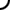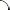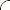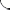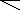Use the abacus worksheet to perform each subtraction operation. Be sure to use commas where appropriate.   57,286 - 12,083 = 43,000 - 14,307 = 18,000 - 13,996 = 45,885 - 16,999 = 985,992 - 343,998 = 257,142 - 251,318 =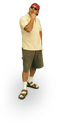Use the abacus worksheet to perform each subtraction operation. Be sure to use commas where appropriate.   74,985 - 13,013 = 31,472 - 26,926 = 46,003 - 23,997 = 47,990 - 15,991 = 158,230 - 145,774 = 463,120 - 321,563 =Use the abacus worksheet to perform each subtraction operation. Be sure to use commas where appropriate.   33,674 - 13,524 = 58,162 - 21,189 = 71,008 - 13,992 = 36,991 - 21,009 = 405,226 - 390,899 = 687,372 - 132,910 =©  2009  LarryPerezWeb Design:  Patrick Quigley